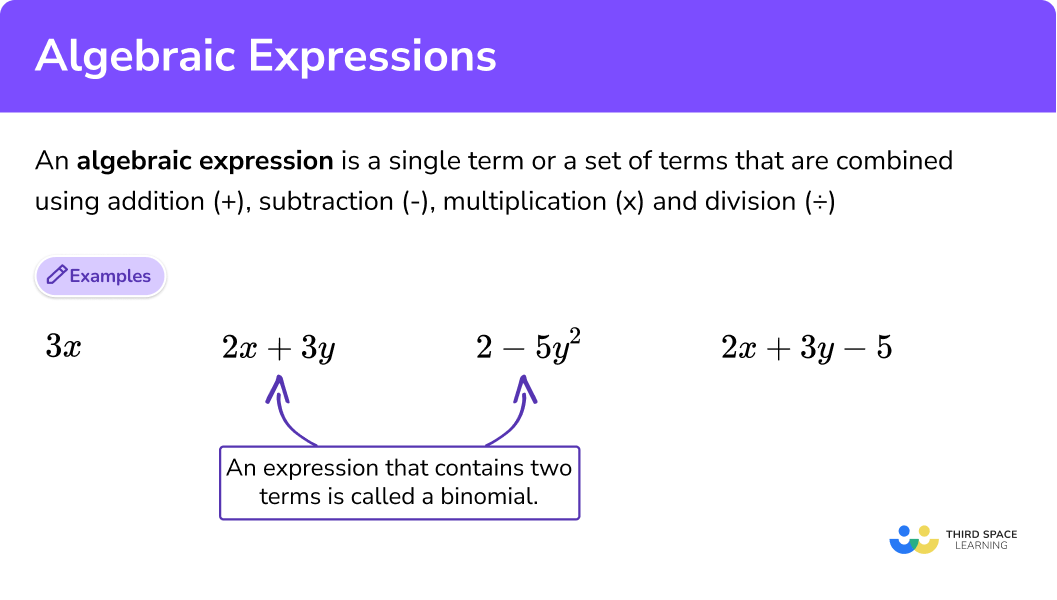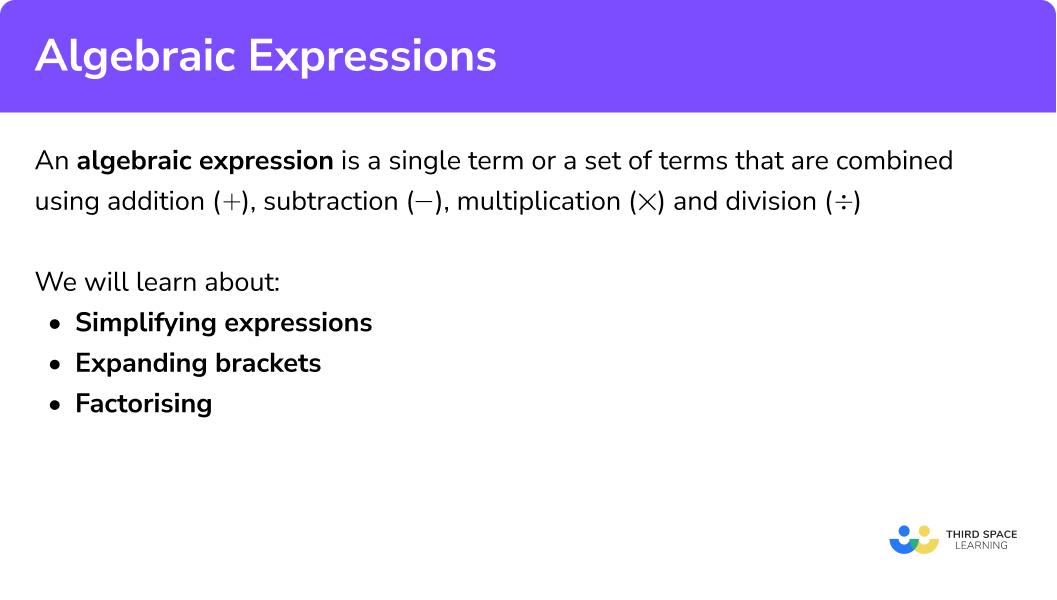GCSE Maths Algebra

Algebraic Expressions

# Algebraic Expressions

Here is everything you need to know about algebraic expressions for GCSE maths (Edexcel, AQA and OCR). You’ll learn what algebraic expressions are, how to simplify algebraic expressions, and the different methods for using algebraic expressions.

Look out for the algebraic expression worksheets, word problems and exam questions at the end.

Overview of this topic: Algebra – Maths GCSE

## What is an algebraic expression?

An algebraic expression is a set of terms with letters and numbers that are combined using addition (+), subtraction (-), multiplication (✕) and division (÷).

An expression that contains two terms is called a binomial.

$E.g. 2x+3y\quad or \quad 2-5y^{2}\quad etc.$

An expression that contains three terms is called a trinomial.

$E.g. 2x+3y-5\quad or \quad 2-5y^{2}+6xy\quad etc.$

### What is an algebraic expression?### Algebraic terms

An algebraic term is either a single number or variable

E.g.  ‘3’ or ‘x’ or ‘z’.

A term can also be a number and a variable multiplied together/

E.g.  ‘2a’ or ‘6y’ or ‘x2’ or ‘4xy’.

When 2 or more algebraic terms are added (or subtracted) they form an algebraic expression.

### Equivalent expressions

Equivalent expressions are expressions which are the same, but look different. For example the expression 3x+4y is equivalent to 4y+3x.

### Keywords

Let’s define some of the keywords when using algebraic notation:

A variable is a symbol (often a letter) that is used to represent an unknown quantity.

$E.g. x\quad or \quad y\quad or\quad a\quad etc.$

Variables can also have exponents (be raised to a certain power).

$E.g. x^{2}\quad or \quad y^{3}$

A coefficient is the value that is before a variable. It tells us how many lots of the variable there is.

\begin{aligned} E.g. 5x&=x+x+x+x+x\\ &=5\times x \end{aligned}

Here 5 is the coefficient and x is the variable.

A term is a number by itself, a variable by itself, or a combination of numbers and letters. If the term includes a variable it is called an algebraic term.

$E.g. 2\quad or\quad 5xy\quad or\quad 12x^{2}\quad or \quad 12xy\quad etc.$

An expression that contains one term is called a monomial.

A polynomial expression consists of two or more algebraic terms.

## How to use algebraic expressions

There are various methods of using algebraic expressions and performing algebraic operations. These are summarised below.

For examples, practice questions and worksheets on each one follow the links to the step by step guides below or go straight to factorising and simplifying expressions.

### What are the methods of using algebraic expressions?### 1. Simplifying expressions

#### a) Collecting like terms

Example of collecting like terms

$5x+3y-3x-7y$

$5 x-3 x=2 x \quad 3 y-7 y=-4 y$

So

$5x+3y-3x-7y = 2x-4y$

#### b) Multiplying and dividing algebra

Example of multiplying and dividing algebra

$5 x y \times 4 x z$
$5 \times 4 = 20$
$x \times x=x^{2}\$
$y \times z=y z$

So

$5 x y \times 4 x z=20 x^{2} y z$

#### c) Expanding brackets

Example of expanding brackets

$3(2x+5)$

Multiply every term outside the bracket by every term inside the bracket.

$3 \times 2 x=6 x \\ 3 \times 5=15 x \\ 3(2x+5)=6x+15$

#### d) Algebraic fractions

Example of algebraic fractions

$\frac{14 x y}{8 x}$

Divide the numerator and the denominator by 2x.

$\frac{7y}{4}$

#### e) Write and simplify algebraic expressions

Example of writing and simplifying algebraic expressions

Write an expression for the perimeter of the shape.

\begin{aligned} Perimeter&=2x+3+x-2+2x+3+x-2\\ &=6x+2 \end{aligned}

### 2. Factorising algebraic expressions

#### a) Factorising to a single bracket

Example of factorising in single brackets

$6 x+3=3(2 x+1)$

#### b) Factorising quadratics – into two brackets

Example of factorising quadratic expressions into two brackets

$2 x^{2}+5 x-3=(2 x-1)(x+3)$

#### c) Difference of two squares

Example of difference of two squares

$4 x^{2}-36=(2 x+6)(2 x-6)$

## Common misconceptions

• Expressions, Equations and Formulas

An algebraic expression is different from an equation and a formula.

An expression is a set of terms that are combined using arithmetic operations:

(+, -, ✕, ÷)

$2x+5$

An equation is an expression that equals something.

$2x+5=15$

We can solve equations to work out the value of the unknown variable (x).

Algebraic formulae are a set of instructions which give a desired result.

E.g. Area of a circle = πr2
Circumference of a Circle = πd

• Brackets terminology

Brackets are sometimes referred to as parentheses.

## Practice algebraic expressions questions

1. Simplify:

6x^{2}y-2x^{2}y+4x^{2}-5x^{2}

10x^{2}y-7x^{2}3x^{2}y10x^{2}y+3x^{2}4x^{2}y-x^{2}By considering like terms in the expression 6x^{2}y-2x^{2}y+4x^{2}-5x^{2} we have 6x^{2}y-2x^{2}y=4x^{2}y and 4x^{2}-5x^{2}=-x^{2} which simplifies to 4x^{2}y-x^{2} .

2. Write an expression for the area of the parallelogram:6x^{2}+17x+56x^{2}+5x+17x+710x+12For the area, we need to multiply the length (3x+1) of the base and the perpendicular height (2x+1) .

Area =(3x+1)(2x+1)=6x^{2}+5x+1

3. Simplify:

\frac{9x^{2}y}{15x^{3}}

\frac{3y}{5x}\frac{9y}{15x}\frac{3x^{2}y}{5x}\frac{3}{5}The numerator and denominator of \frac{9x^{2}y}{15x^{3}} have a highest common factor of 3x^{2} . Therefore, we divide through by 3x^{2} resulting in the simplified algebraic fraction.

4. Simplify:

\frac{x^{2}-3x-10}{x^2-25}

\frac{x-5}{x-5}\frac{x+2}{x-5}\frac{x+2}{x+5}x^{2}-3x-35The numerator can be factorised as the product of two brackets

x^{2}-3x-10=(x+2)(x-5)

The denominator can be factorised as it is the difference of two squares

x^{2}-25=(x+5)(x-5)

This means we can write the fraction as

\frac{(x+2)(x-5)}{(x+5)(x-5)}

The numerator and denominator have a common factor of x-5 , which we cancel, leaving the simplified fraction.

5. Simplify:

3x(4-5x+2y)

12-15x+6y12x-15x^{2}+6xy7x-9x^{2}+5xy12x-15x+6yWe need to multiply each term inside the bracket by 3x

3x\times4=12x\\ 3x\times(-5x)=-15x^{2}\\ 3x\times2y=6xy

which we can combine into the expression we need as required.

6. Expand and simplify:

4(2x-1)-3(x+6)

5x-2211x-22x-511x+14We can expand each bracket one at a time

4(2x-1)=8x-4

and

-3(x+6)=-3x-18

This can be further simplified by collecting like terms

8x-4-3x-18=5x-22

7. Fully factorise:

2{x}^2+x-6

(2 x+2)(x-3)2\left(x^{2}+0.5 x-3\right)(2 x-3)(x+2)(x-3)(x+2)=(2x-3)(x+2)

To factorise the quadratic expression, we are looking for numbers that multiply to -12 and sum to +1. By considering factor pairs, we conclude that we need to use +4 and -3.

We can rewrite

2x^{2}+x-6

as 2x^{2}+4x-3x-6

which can be factorised as

2x(x+2)-3(x+2)

or more concisely

(2x-3)(x+2)

8. Fully factorise:

4{x}^2-25

(2 x+5)(2 x-5) \\(2 x-5)(2 x-5) \\(4 x+5)(x-5) \\(3 x+20)(x+5)(2 x+5)(2 x-5)

This is a special case (difference of two squares), which means we can take square roots of the coefficient of x and the constant term, then write one bracket with a + sign and the other bracket with a – sign.

## Algebraic expressions GCSE questions

1. Simplify: 4f – 2e + 3f + 5e

7f + 3e

(2 marks)

2. Expand and simplify: 4x(2x – 7)

8x2 – 28x

(2 marks)

3. Simplify:

$\frac{15x^{3}y^{2}}{5xy^{3}}$

$=\frac{3x^{2}}{y}$

(2 marks)

## Learning checklist

• simplify expressions
• use language and properties precisely to analyse algebraic expressions
• simplify and manipulate algebraic expressions to maintain equivalence by:
– collecting like terms
– multiplying a single term over a bracket
– taking out common factors
• translate simple situations or procedures into algebraic expressions

## Still stuck?

Prepare your KS4 students for maths GCSEs success with Third Space Learning. Weekly online one to one GCSE maths revision lessons delivered by expert maths tutors.

Find out more about our GCSE maths tuition programme.